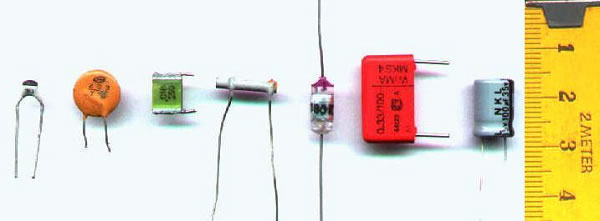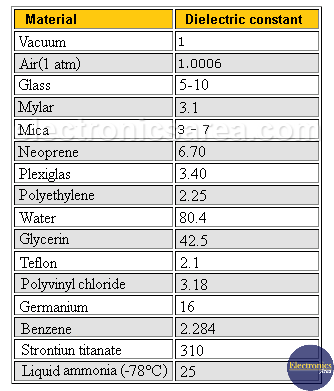# Dielectric Constant / Relative Permittivity

The dielectric constant or Relative Permittivity is a dimensionless physical constant (Dielectric constant has no units) that describes how an electric field affects a material. The dielectric constant is the ratio of the permittivity of a substance to the permittivity of free space.

Capacity of a capacitor depends on the dielectric constant. It is known that the value of the capacity of a capacitor is given by the following formula: C = Q / V. Where:

• C: Capacitor capacity
• Q: capacitor charge
• V: Potential difference (voltage) between the capacitor platesThe capacitance of a capacitor can also be obtained differently by including the value of the dielectric constant.

## How to obtain the Capacity knowing the dielectric constant and its physical dimensions?

A capacitor is formed by two parallel plates. If there is a vacuum between these plates, the value of the capacity is: C = εo a / d. where:

• a = area of ​​each plate in m2
• d = distance between plates in meters
• εo = dielectric constant in vacuum, whose value is: 8.85 x 10-12 farad / meter

If a dielectric is introduced between the plates, the capacitance will increase by a factor εr. So the capacity is:
C = εo εr a / d or C = ε a / d, where:

• ε = εo εr
• εr is the relative dielectric constant and depends on the physical properties of the medium used.
• ε is the absolute dielectric constant.

There is a great difference between values ​​of the dielectric constants of different materials. Some important examples of dielectric constants are shown in the following table.

## Table of dielectric constantsTable of dielectric constants (20 °C)

Example of capacity and charge calculation of parallel plate’s capacitor.

We have a parallel plates capacitor separated by vacuum. The plates are 1 mm apart and have an area of ​​2 x 10-6 meters. If the capacitor is connected to a voltage of 250 volts:

1. What is the capacitance of the capacitor?
2. What is the charge on each plate?
3. What is the capacitance be if it has a paper dielectric?

1. Capacitance:
Using the formula: C = εo εr a / d.
C = (8.85 x 10-12) (1) (2 x 10-6) / (1 x 10-3) = 1.77 x 10-14 Farads.

2. Charge on each plate:
Using the formula: C = Q / V, we obtain that Q = C x V.
Q = (1.77 x 10-14) x 250 = 4.425 x 10-12 Coulombs.

3. Capacitance with paper dielectric (εr = 2.5)
Using the formula: C = εo εr a / d
C = (8.85 x 10-12) (2.5) (2 x 10-6) / (1 x 10-3) = 4.425 x 10-14 Farads.

You can see the increase in capacitor capacity with a higher value dielectric.

•
•
• 2
•
•
•
•
•
2
Shares
•
2
Shares
•
•
• 2
•
•
•
•